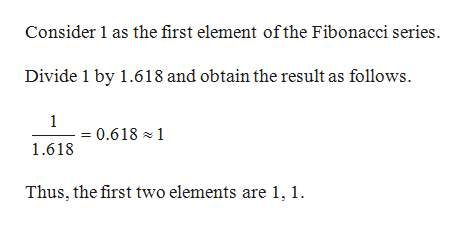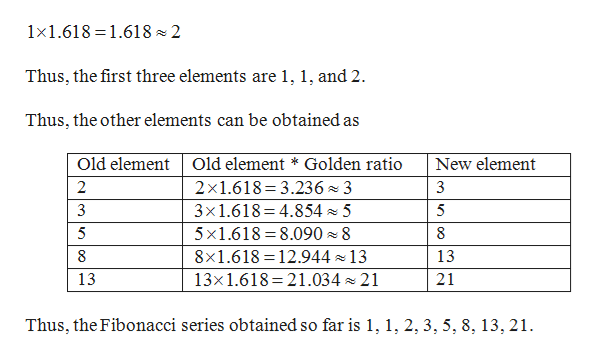How do i calculate the fibonacci sequence in relation to the golden ratio?

Question

How do i calculate the fibonacci sequence in relation to the golden ratio?

Step 1

The golden ratio is a unique number whose value is 1.618.help_outlineImage TranscriptioncloseConsider 1 as the first element ofthe Fibonacci series. Divide 1 by 1.618 and obtain the result as follows. 1 = 0.618 1 1.618 Thus, the first two elements are 1, 1. fullscreen
Step 2

In order to obtain the third element, mul...help_outlineImage Transcriptionclose1x1.618 1.618 2 Thus, the first three elements are 1, 1, and 2 Thus, the other elements can be obtained as Old element Old element * Golden ratio New element 2x1.618 3.236 3 3x1.618 4.8545 5 x1.618 8.090 8 8x1.618 12.944 13 13x1.618 21.03421 2 3 5 5 8 8 13 21 13 Thus, the Fibonacci series obtained so far is 1, 1, 2, 3, 5, 8,13, 21 fullscreen

Want to see the full answer?

See Solution

Want to see this answer and more?

Our solutions are written by experts, many with advanced degrees, and available 24/7

See Solution
Tagged in

Math